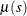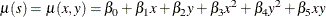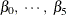# The SIM2D Procedure

### MEAN Statement

MEAN spec1,, spec6 ;

MEAN QDATA=SAS-data-set CONST=var1 CX=var2 CY=var3 CXX=var4 CYY=var5 CXY=var6 ;

MEAN QDATA=SAS-data-set ;

A mean functionthat is a quadratic in the coordinates can be written asThe MEAN statement specifies the quadratic surface to use as the mean function for the simulated SRF. There are two ways to specify the MEAN statement. The MEAN statement allows the specification of the coefficientseither explicitly or through a QDATA= data set.

An example of an explicit specification is the following:

mean 1.4 + 2.5*x + 3.6*y + 0.47*x*x + 0.58*y*y + 0.69*x*y;


In this example, all terms have a nonzero coefficient. Any term with a zero coefficient is simply left out of the specification. For example,

mean 1.4;


is a valid quadratic form with all terms having zero coefficients except the constant term.

An equivalent way of specifying the mean function is through the QDATA= data set. For example, the MEAN statement

mean 1.4 + 2.5*x + 3.6*y + 0.47*x*x + 0.58*y*y + 0.69*x*y;


can be alternatively specified by the following DATA step and MEAN statement:

data q1;
input c1 c2 c3 c4 c5 c6;
datalines;
1.4 2.5 3.6 0.47 0.58 0.69
;

proc sim2d data=....;
simulate ...;
mean qdata=q1 const=c1 cx=c2 cy=c3 cxx=c4 cyy=c5 cxy=c6;
run;


The QDATA= data set specifies the data set containing the coefficients. The parameters CONST=, CX=, CY=, CXX=, CYY=, and CYX= specify the variables in the QDATA= data set that correspond to the constant, linear y, linear y, and so on. For any coefficient not specified in this list, the QDATA= data set is checked for the presence of variables with default names of CONST, CX, CY, CXX, CYY, and CXY. If these variables are present, their values are taken as the corresponding coefficients. Hence, you can rewrite the previous example as follows:

data q1;
input const cx cy cxx cyy cxy;
datalines;
1.4 2.5 3.6 0.47 0.58 0.69
;

proc sim2d data=....;
simulate ...;
mean qdata=q1;
run;


If a given coefficient does not appear in the list or in the data set with the default name, a value of zero is assumed.

If you run a simulation task with input from a RESTORE statement, then by default the simulation uses the mean of the item store variable in the simulation. You can override this default behavior if you explicitly specify the MEAN statement with a different mean function.